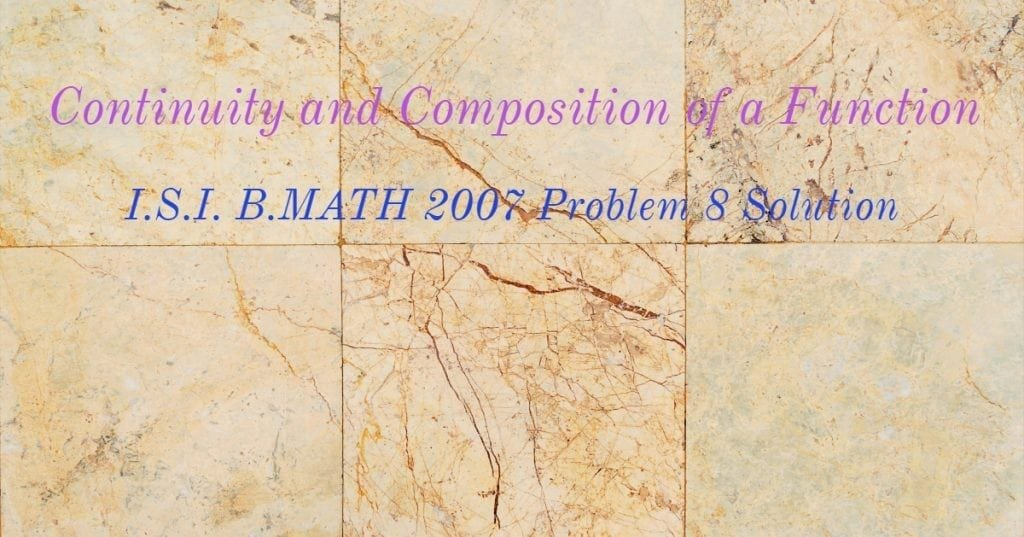How Cheenta works to ensure student success?
Explore the Back-Story

# Continuity and composition of a function | ISI BMath 2007This is a problem number 8 from ISI BMath 2007 based on the Continuity and composition of a function. Try this out.

Problem: Continuity and composition of a function

Let $\mathbf{P:\mathbb{R} \to \mathbb{R}}$ be a continuous function such that $P(x)=x$ has no real solution. Prove that $P(P(x))=x$ has no real solution.

Discussion:

Hunch: There is no solution of $P(x) = x$ implies the graph of $P(x)$ never 'crosses' the $y=x$ line,
Suppose $P(P(x)) = x$ has a solution at $x = a$ then $P(P(a)) = a$.

Suppose $P(a) = b$ (b is not equal to a as P(x) = x has no solution) then $P(P(a)) = a$ implies $P(b) = a$. Hence we have the following:
$P(a) = b$ and $P(b) = a$.

There fore the points $(a, b)$ and $(b, a)$ are both on the graph of $P(x)$. But these two points are reflections about $y=x$ line implying $P(x)$ is on both side of that line hence continuity implies $P(x)$ must intersect $y=x$ line leading to a solution of $P(x) = x$ hence contradiction. Therefore $P(P(x)) = x$ has not solution.

Final Solution

Consider the auxiliary function $g(x) = P(x) - x$. If $P(P(x)) = x$ has a solution then we have already established that there exists $a, b$ such that $P(a) = b$ and $P(b) = a$. We plug in $a, b$ in $g(x)$.

$g(a) = P(a) - a = b - a$
$g(b) = P(b) - b = a - b$

Now one of $a-b$ and $b-a$ is positive. The other is negative. Since $P(x)$ is continuous so is $g(x)$. So by intermediate value property theorem, $g(x)$ will become $0$ for some value $c$ between $a$ and $b$.

$g(c) = 0$ implies $P(c) - c = 0$ or $P(c) = c$ a contradiction.

Hence $P(P(x)) = x$ has no solution.

Proved

Our ISI CMI Program

How to use invariance in Combinatorics – ISI Entrance Problem – Video

This is a problem number 8 from ISI BMath 2007 based on the Continuity and composition of a function. Try this out.

Problem: Continuity and composition of a function

Let $\mathbf{P:\mathbb{R} \to \mathbb{R}}$ be a continuous function such that $P(x)=x$ has no real solution. Prove that $P(P(x))=x$ has no real solution.

Discussion:

Hunch: There is no solution of $P(x) = x$ implies the graph of $P(x)$ never 'crosses' the $y=x$ line,
Suppose $P(P(x)) = x$ has a solution at $x = a$ then $P(P(a)) = a$.

Suppose $P(a) = b$ (b is not equal to a as P(x) = x has no solution) then $P(P(a)) = a$ implies $P(b) = a$. Hence we have the following:
$P(a) = b$ and $P(b) = a$.

There fore the points $(a, b)$ and $(b, a)$ are both on the graph of $P(x)$. But these two points are reflections about $y=x$ line implying $P(x)$ is on both side of that line hence continuity implies $P(x)$ must intersect $y=x$ line leading to a solution of $P(x) = x$ hence contradiction. Therefore $P(P(x)) = x$ has not solution.

Final Solution

Consider the auxiliary function $g(x) = P(x) - x$. If $P(P(x)) = x$ has a solution then we have already established that there exists $a, b$ such that $P(a) = b$ and $P(b) = a$. We plug in $a, b$ in $g(x)$.

$g(a) = P(a) - a = b - a$
$g(b) = P(b) - b = a - b$

Now one of $a-b$ and $b-a$ is positive. The other is negative. Since $P(x)$ is continuous so is $g(x)$. So by intermediate value property theorem, $g(x)$ will become $0$ for some value $c$ between $a$ and $b$.

$g(c) = 0$ implies $P(c) - c = 0$ or $P(c) = c$ a contradiction.

Hence $P(P(x)) = x$ has no solution.

Proved

Our ISI CMI Program

How to use invariance in Combinatorics – ISI Entrance Problem – Video

This site uses Akismet to reduce spam. Learn how your comment data is processed.

### One comment on “Continuity and composition of a function | ISI BMath 2007”

1. […] Let be a continuous function such that P(X)=X has no real solution. Prove that P(P(X))=X has no real solution.Solution […]

### Knowledge Partner